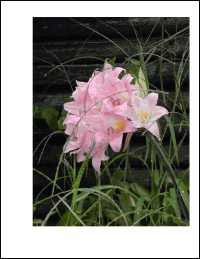Image ExampleThis example shows how to create a simple PDF displaying an image.Image

 First, we create an ABCpdf Image object and we assign our image file. ```Set theImg = Server.CreateObject("ABCpdf12.Image") theImg.SetFile "c:\mypics\pic.jpg"```Doc

 Next, we create an ABCpdf Doc object. When we add our image, it will be scaled to fit the current rect so it is important that we adjust the rect to reflect the dimensions of our image. Here, we assume a one to one ratio between pixels and points, which will give us a 72 dpi result when printed. ```Set theDoc = Server.CreateObject("ABCpdf12.Doc") theDoc.Rect.Left = 100 theDoc.Rect.Bottom = 100 theDoc.Rect.Width = theImg.Width theDoc.Rect.Height = theImg.Height theDoc.AddImageObject theImg, False theDoc.Save "c:\mypdfs\image.pdf"```Resultsimage.pdf